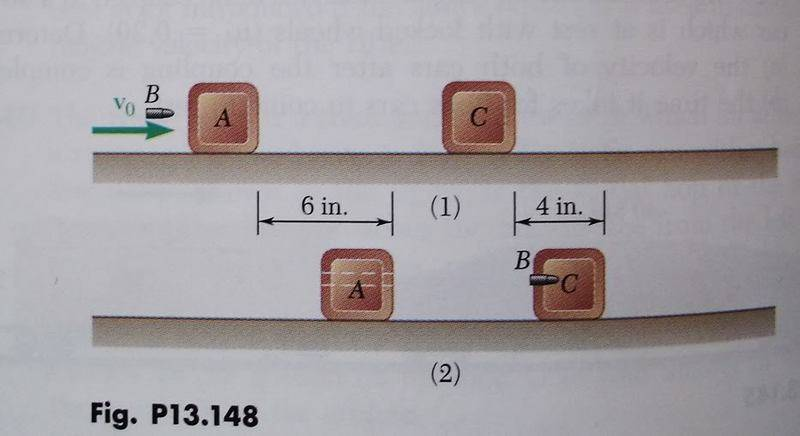# Impulse - Initial velocity of bullet

xicor
1. Homework Statement [/b]

Bullet B weighs 0.5 oz and blocks A and C both weigh 3 lb. The coeffient of friction between the block and the plane is $$\mu$$k = .25. Initially the bullet is moving at v0 and blocks A and B are at rest. After the bullet passes through A it becomes embedded in block C and all three objects come to stop in the position shown. Determine the initial speed of the bullet v0.mv1 + Imp(1 $$\rightarrow$$ 2) = mv2

Imp(1 $$\rightarrow$$ 2) = int[F(t)dt]

U(1 --> 2) = T2 - T1 where T is kinetic energy and U is potential energy

U(1-->2) = int[F.dr]

## The Attempt at a Solution

I'm not really sure where to actually start with the problem besides starting from the second block. I tried using the impulse priniciple but that gives me an unknown time, force, and velocity and I'm not sure if the force would just be the fictional force. I also tried appying the work energy theorem but I'm not confident of that being useful here and it ended up giving me a huge velocity.

Thanks to anybody that is willing to helps me.

Last edited:

Homework Helper
Hi xicor!… I also tried appying the work energy theorem but I'm not confident of that being useful here and it ended up giving me a huge velocity.

That should do fine… the work done should give you the initial speed of each block.

What did you get?​

xicor
Alright I used the work energy theorem on the second block and got v1^2 = $$\sqrt{(2/3 )(32.2)}$$ --> v1/ =4.633 ft/s which I'm not sure is right but it seems to be. After that I than tried to find the speed of the bullet between the two blocks by conservation of momentum(m1v1 =m2v2) nd found the v = 449.4 ft/s. Once I found this value I used the W.E.T on the first block where T1 is the kinetic energy of the block when hit by the bullet and T2 is the kinetic energy of the bullet after going through the block. From W.E.T I get the initial velocity of the first block to be 45.5 ft/s which seems way to big and using linear momentum again I get the initial velocity of the bullet as 4413.6 ft/s which is way to big a velocity in the problem.

I dont know where I'm going wrong here.

Last edited:
Hi xicor!No, you haven't used µ = 0.25 in finding the work done on the block.ii] then write out the two separate conservation of momentum equations for the two separate collisions